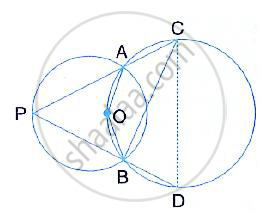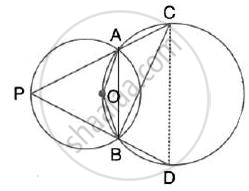Share

In the Given Figure, the Centre O of the Small Circle Lies on the Circumference of the Bigger Circle. If ∠Apb = 75° and ∠Bcd = 40°, Find: (I) ∠Aob, (Ii) ∠Acb, (Iii) ∠Abd, (Iv) ∠Adb - ICSE Class 10 - Mathematics

ConceptArc and Chord Properties - the Angle that an Arc of a Circle Subtends at the Center is Double that Which It Subtends at Any Point on the Remaining Part of the Circle

Question

In the given figure, the centre O of the small circle lies on the circumference of the bigger circle. If ∠APB = 75° and ∠BCD = 40°, find:
(i) ∠AOB, (ii) ∠ACB, (iii) ∠ABD, (iv) ∠ADBSolutionJoin AB and AD
(i) ∠AOB = 2 ∠APB = 2 ×75° =150°
(Angle at the centre is double the angle at the circumference subtended by the same chord)

(ii) In cyclic quadrilateral AOBC,
∠ACB =180° - ∠AOB = 180° -150° = 30°
(pair of opposite angles in a cyclic quadrilateral are supplementary)

(iii) In cyclic quadrilateral ABDC
∠ABD = 180° - ∠ACD = 180° - (40° + 30° ) = 110°
(pair of opposite angles in a cyclic quadrilateral are supplementary)

(iv) In cyclic quadrilateral AOBD,
∠ADB = 180° - ∠AOB = 180° -150° = 30°
(pair of opposite angles in a cyclic quadrilateral are supplementary)

Is there an error in this question or solution?

Video TutorialsVIEW ALL 

Solution In the Given Figure, the Centre O of the Small Circle Lies on the Circumference of the Bigger Circle. If ∠Apb = 75° and ∠Bcd = 40°, Find: (I) ∠Aob, (Ii) ∠Acb, (Iii) ∠Abd, (Iv) ∠Adb Concept: Arc and Chord Properties - the Angle that an Arc of a Circle Subtends at the Center is Double that Which It Subtends at Any Point on the Remaining Part of the Circle.
S